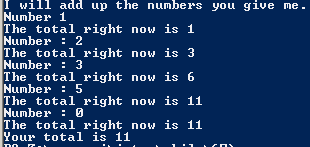# Assignemnt #67

## Code

```///colin hinton
///5th period
///11/6/2015

import java.util.Scanner;

{
public static void main (String [] args)
{
Scanner keyboard = new Scanner(System.in);
int total;

System.out.println("I will add up the numbers you give me.");
System.out.print("Number ");
int x = keyboard.nextInt();
total = x;
System.out.println("The total right now is " + total);

while (x != 0)
{
System.out.print("Number : ");
x = keyboard.nextInt();
total = (x + total);
System.out.println("The total right now is " + total);
}

System.out.println("Your total is " + total);
}
}

```

### Picture of the output